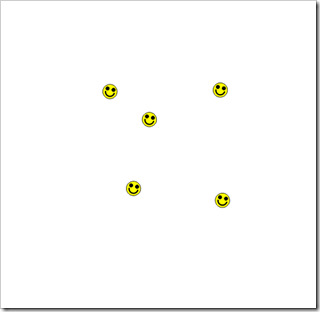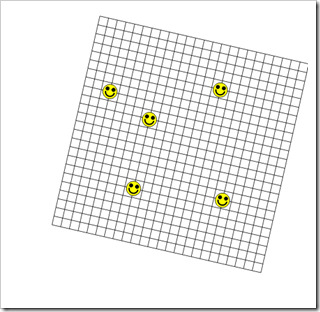I could not come up with a simple math solution for this. By “simple math”, I mean a math solution that uses a single equation without sine-cosine operations to solve the problem.

### The Problem

I have an image that is a picture of a grid of information. The picture is a photo and may have the grid visible at any size and any rotation.##### The Picture

Additionally, the grid information may be hard to see in some places due to the nature of the content.

The user needs to open the image and click in two places in the image and then tell the program about the two clicked locations. They tell the program the x,y coordinates of the two places within the grid and the program already knows the x,y coordinates within the image.

I need to determine the location, size, and orientation of the grid and draw it on top of the image. This needs to work for any image with any grid size, location, and orientation.

### The Solution (Using Geometric Stuff)

First the user needs to click somewhere to solve this problem. The first click is on the left-most smiley face. The user then tells the program that this is at location 3,8 in the grid. Then they click on the bottom-right smiley face and tell the program that it is at location 18,17 in the grid.

Assuming that the upper left corner of the grid is 0,0 and the upper left pixel in the image is 0,0, these are the steps to find the grid location using data from within the grid:

1. Calculate the distance in pixels and in grid spaces between the two smiley faces. This is the distance directly, not the x,y distances. This tells us how many pixels are in each step of the grid or a ratio of pixels to grid units, whatever you want to call it.
2. Get the angle from the left smiley face to the bottom-right one relative to the grid.
3. Get the angle from the left smiley face to the bottom-right one relative to the pixels in the image.
4. Get the difference between the two angles.
5. Rotate the coordinates of the right smiley face by the angle difference from step 4. The right smiley face is now in the correct location as if the grid were not rotated relative to the pixels.
6. Now the interesting part. Setting DX=grid_to_pixel_count and DY=0, rotate DX,DY around 0,0 using the negated angle from step 4. The result is a delta for X and a delta for Y for stepping one column right in the grid. These values can be switched and negated properly to step one row in the grid too.
7. Start at the first selected smiley face and then step -4 columns, the negated user-specified grid column of the first smiley face, using DX and DY.
8. Step -8 rows using DX and DY, -DY is used for the X coordinate change and –DX is used for the Y coordinate change (I think that I write that correctly).
9. The upper left corner of the grid is found. The DX and DY stepping values are known for the grid so it can now be drawn under the smiley faces to show the result.##### The Resulting Grid Underlay

My daughter and I tried to come up with a math solution that does not use angles and rotate points. It might be possible using ratios of the various numbers but we could not find the solution. Rotating one point and using it to get values that are then rotated the opposite way is not a bad solution and it works great.